Function Repository Resource:

# Equisample

Sample evenly spaced elements from a list

Contributed by: Michael Sollami
 ResourceFunction["Equisample"][list] chooses equally spaced elements from list. ResourceFunction["Equisample"][list,n] chooses n equally spaced elements. ResourceFunction["Equisample"][list,Scaled[m/n]] chooses m out of every n equally spaced elements.

## Details and Options

Without providing a second argument, ResourceFunction["Equisample"] will return a number of samples logarithmic in the length of its list argument.

## Examples

### Basic Examples (3)

Sample 10 evenly spaced elements from a range:

 In:=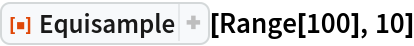Out=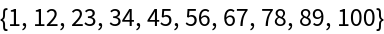In:=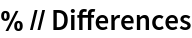Out=Sample one of every five elements:

 In:=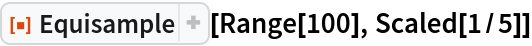Out=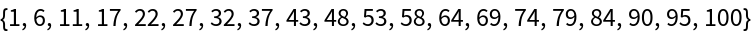Take default numbers of equi-spaced samples:

 In:=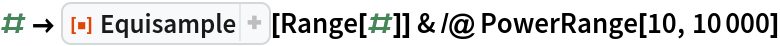Out=### Possible Issues (1)

When the requested samples cannot be exactly equally-spaced, Equisample returns (similar to FindDivisions) a "nice" approximation:

 In:=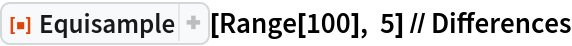Out=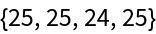Michael Sollami

## Version History

• 1.0.0 – 06 January 2020